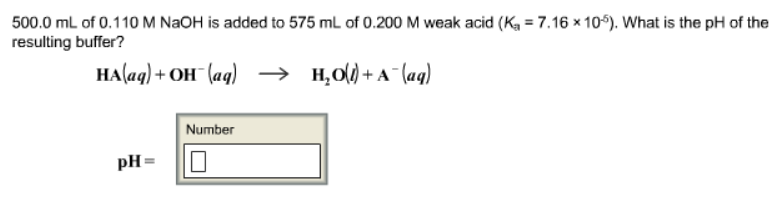# Problem: 500.0 mL of 0.110 M NaOH is added to 575 mL of 0.200 M weak acid (K   a = 7.16 x 10-6). What is the pH of the resulting buffer? HA (aq) + OH - (aq) → H2O (l) + A- (aq)

###### FREE Expert Solution
96% (167 ratings)###### Problem Details

500.0 mL of 0.110 M NaOH is added to 575 mL of 0.200 M weak acid (K   a = 7.16 x 10-6). What is the pH of the resulting buffer?

HA (aq) + OH (aq) → H2O (l) + A(aq)